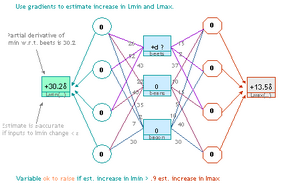## neal young / Young01Sequential

•Mixed packing and covering problems are problems that can be formulated as linear programs using only non-negative coefficients. Examples include multicommodity network flow, the Held-Karp lower bound on TSP, fractional relaxations of set cover, bin-packing, knapsack, scheduling problems, minimum-weight triangulation, etc. This paper gives approximation algorithms for the general class of problems. The sequential algorithm can be implemented to find an $$(1\pm\epsilon)$$-approximate solution in $$O(\epsilon^{-2}\log m)$$ linear-time iterations.

For $$\epsilon = O(1)$$, these algorithms are currently the fastest known for the general problem. The results generalize previous work on pure packing and covering (the special case when the constraints are all less-than'' or all greater-than'') by Michael Luby and Noam Nisan ; and Naveen Garg and Jochen Konemann ; and Lisa Fleischer